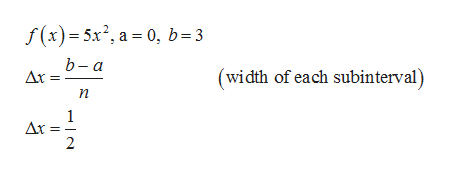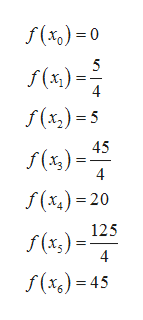# Use the trapezoidal rule and Simpson's rule to approximate the value of the definite integral. Compare your result with the exact value of the integral. (Give your answers correct to 4 decimal places.)0∫35x2dx; n=6

Question
2 views

Use the trapezoidal rule and Simpson's rule to approximate the value of the definite integral. Compare your result with the exact value of the integral. (Give your answers correct to 4 decimal places.)

035x2dx; n=6
check_circle

Step 1

The given integral is,

Step 2

The function, f with the limits and width of each subintervals are,help_outlineImage Transcriptionclosef(x)5x2, a 0, b=3 b-а Ax (width of each subinterval) п 1 Ar = 2 - fullscreen
Step 3

Compute the values of the function, a...help_outlineImage Transcriptionclosef(x) 0 5 f(x)= 4 f(x)s 45 f() 4 f(x)20 125 f (x) 4 f() 45 fullscreen

### Want to see the full answer?

See Solution

#### Want to see this answer and more?

Solutions are written by subject experts who are available 24/7. Questions are typically answered within 1 hour.*

See Solution
*Response times may vary by subject and question.
Tagged in

### Calculus